# 月之暗面

The DARKSIDE of the Moon

0%

# 基础模拟与数字调制技术

sorry,我仔细看了那篇教程后发现其并不是特别适合写这篇文。那么我就从Wiki和各种参考书和教材上搜集资料了。

# Working List

• AM
• FM
• PM
• FSK
• PSK
• 特殊信号
• 高阶调制 鸽鸽还在咕😭
• 其他 s # 为什么要调制？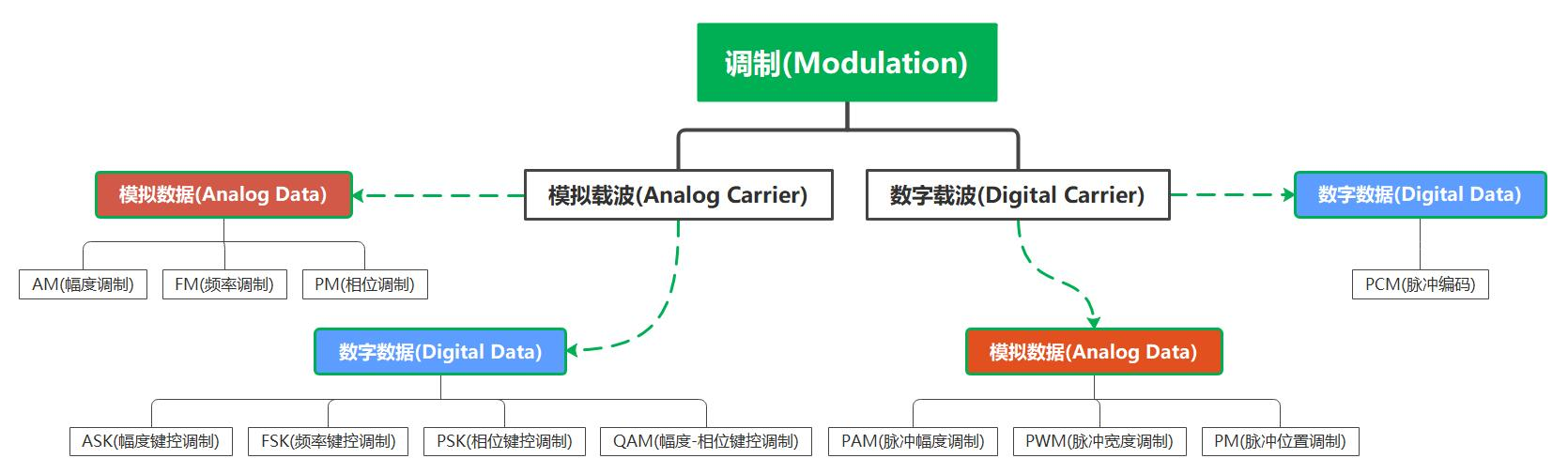Name English 意义

# 一.模拟调制

## 1.1 AM(Amtitude Modulation)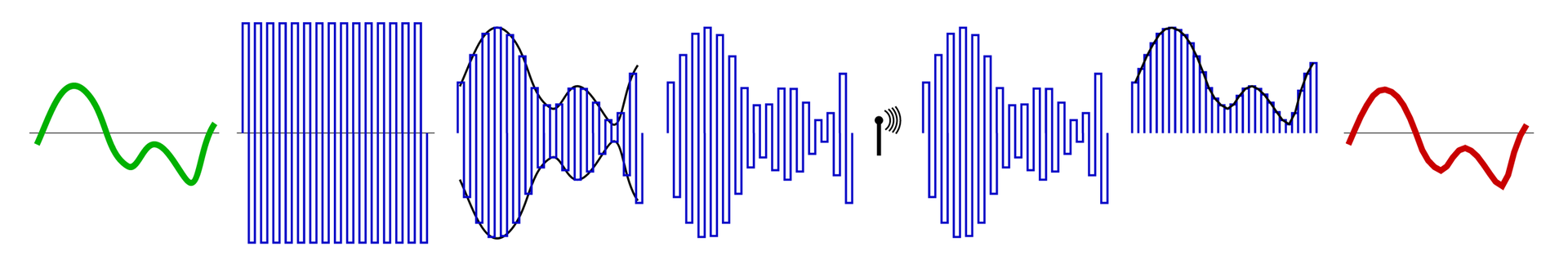### 1.1.1 数学形式

$c(t) = A\sin(2\pi f_c t)$

$$m(t)$$ 表示调制波形(基带信号),载波的频率比基带的频率要高得多:

$m(t) = M·\cos(2\pi f_mt + \phi)$

$y(t) = [1+m(t)]·c(t) \\ = [1+M·cos(2\pi f_m t + \phi)]·Asin(2\pi f_c t)$

$y(t) = A·\sin(2\pi f_ct) + {AM \over 2}[\sin(2\pi(f_c+f_m)t + \phi) + \sin(2\pi(f_c-f_m)t - \phi)]$

- 频率略高于载波频率的 上边带(upper-band)
- 频率略低于载波频率的 下边带(lower-band)### 1.1.2 调制指数与失真

$m = {m(t)的峰值 \over A}={M \over A}$import matplotlib as mpl
import matplotlib.pyplot as plt
import numpy as np
from cycler import cycler

N = 4000			# 采样点4000点
f_baseband = 1e2	# 包络频率100Hz
f_carrier = 1e3		# 载波频率10kHz

# 时间间隔
t = np.linspace(-2/f_baseband, 2/f_baseband, num=N)
# 生成包络与载波信号
baseband = np.sin(2 * np.pi * t * f_baseband)
carrier = np.sin(2 * np.pi * t * f_carrier)
# 调制指数m
m = 1.0

# Plot
fig, axd = plt.subplot_mosaic([['upleft', 'right'],
['lowleft', 'right']], layout='constrained')
axd['upleft'].set_title('baseband signal')
axd['upleft'].plot(t, baseband, 'k')

axd['lowleft'].set_title('Carrier signal')
axd['lowleft'].plot(t, carrier, 'k')

axd['right'].set_title('Modulated signal')
axd['right'].plot(t, (1+m*baseband)*carrier, 'k')
axd['right'].legend()
# show
plt.show()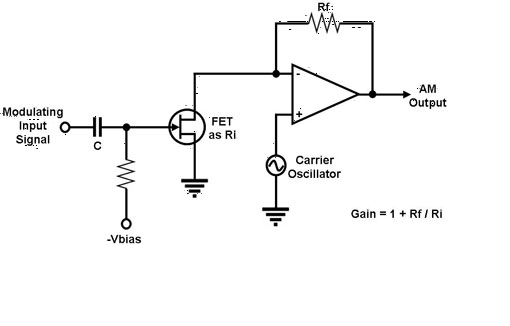### 1.1.3 AM的优缺点

1. 浪费功率(效率低)
载波 $$c(t)$$ 与带有信息的基频信号 $$m(t)$$ 并不相关。因此，传输载波就会浪费功率，也就是说，在调幅的总功率中，有一部分是真正有被 $$m(t)$$ 影响，有另一部分是被浪费的。
2. 浪费带宽(频带利用率低)
AM波的上边带及下边带相互对称于载波频率，所以若我们知道其中一组边带的幅度谱及相位谱，由于对称性，我们就可以知道另外一组。这也表示在信息传输的时候，其实只有一组边带就够了，因此信道也只需要提供等同于基频信号的带宽，这样来看，因为调幅需要的传输带宽是信息带宽的两倍，所以我们可以称它是 浪费带宽的。

### 1.1.4 AMの超进化

1. 双边带抑制载波调制(DSB-SC,=Double-sided band-Suppressed carrier) DSB-SC的发射波只由上下边带组成。因为抑制了载波，所以可以节省传输功率，但是信道带宽需求还是跟原来一样，也就是信息带宽的两倍。
2. 残留边带调制(VSB, =Vestigial-Sided band) VSB调制让一组边带几乎全部通过，而另一组边带几乎全不通过，也就是有少量的残留。所以，VSB的带宽需求，比起信息带宽多了前述的残留边带的宽度。这种方式的调制，很适合用在宽频信号，比如说电视信号，在极低频率处含有显著的成分。又例如说，在电视广播中，很强的载波会跟被调波一起被发送，所以我们能在接收端处用包络检波器来解调信号，因此接收器的设计得以被简化。
3. 单边带调制(SSB,=Single-Sided band) SSB的被调波只由上边带或者是下边带组成，所以，SSB调制的功能是，将调制波的频谱在频域中转移到新的位置。单边带调制适合传送声音频号。这样的调制方法需要的传输功率以及信道带宽都最小，但缺点是实现成本的增加以及系统复杂度的增加(哪种改进都得增加orz)。

## 1.2 FM(Frequency Modulation)

AM和FM的区别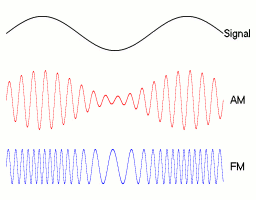### 1.2.1 数学形式

$y(t) = A_c\cos(2\pi \int_0^t f(\tau) d\tau) \\ = A_c\cos(2\pi \int_0^t [f_c+f_\Delta x_m(\tau)] d\tau) \\ = A_c\cos(2\pi f_ct + 2\pi f_\Delta \int_0^t x_m(\tau)d\tau)$

$\int_0^t x_m(\tau) d\tau = {\sin(2\pi f_mt) \over 2\pi f_m}$

$y(t) = A_c\cos(2\pi f_ct + {f_\Delta \over f_m} \sin(2\pi f_mt))$

FM信号的频谱图(上部)+瀑布图(下部)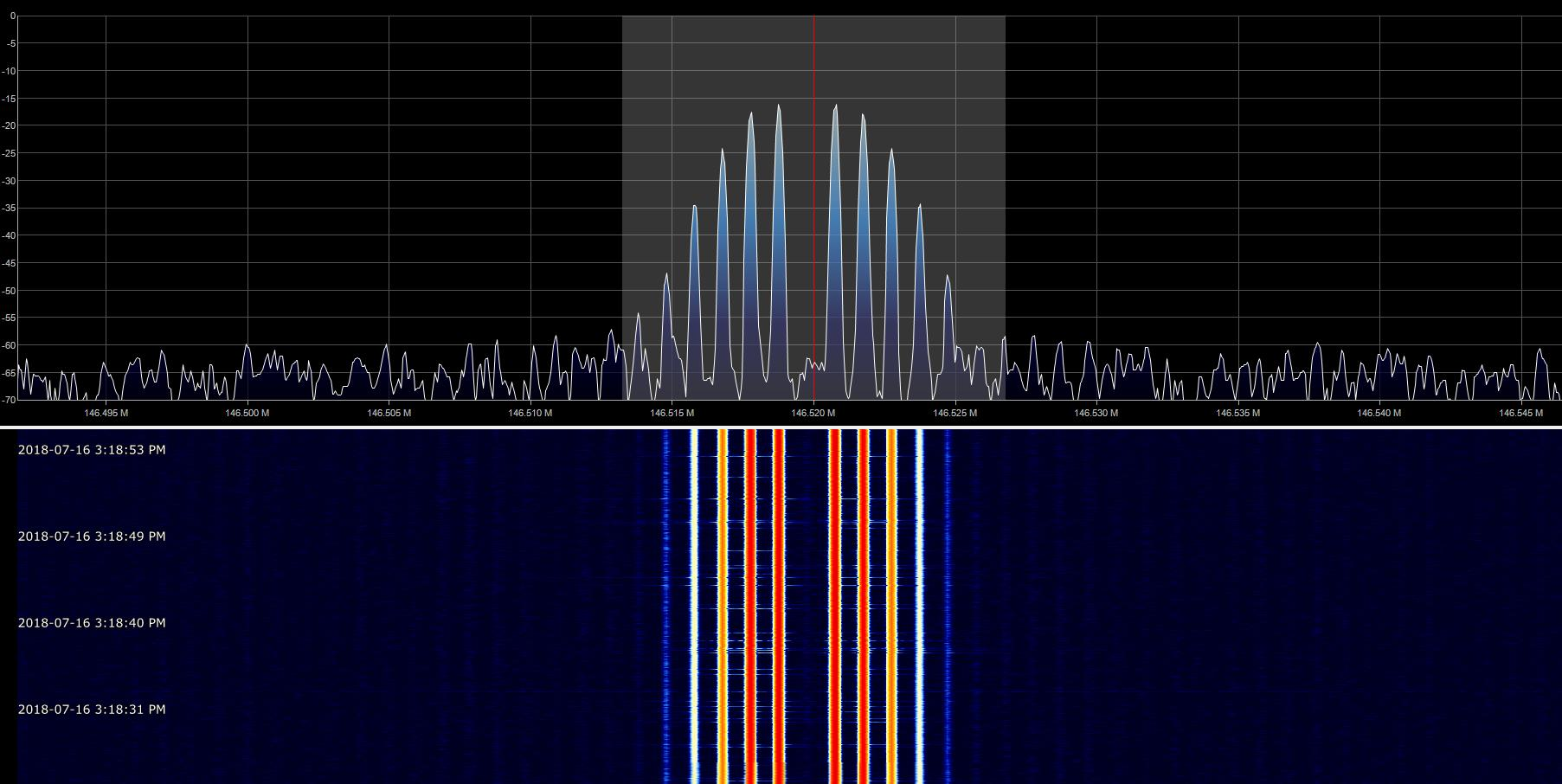### 1.2.2 调制指数

$h = {\Delta f \over f_m} = {f_\Delta |x_m(t)| \over f_m}$

$$h\ll 1$$,则该调制称为 窄带调频(Narrow-Band FM, NFM),而其带宽约为 $$2f_m$$。有时 $$h \lt 0.3$$ 被视为NFM,否则就是 宽带(Wide-Band FM, WFM)调频

### 1.2.3 卡森法则(Carson‘s rule)

Carson's rule是一个经验法则,它指出了几乎所有的调频信号的功率 分布在带宽 $$B_T$$内,这个带宽被称为 卡森带宽:

$B_T = 2(\Delta f + f_m) = 2f_m(\beta + 1)$

$B_T = 2(\Delta f + W) = 2W(D + 1)$

$$W$$ 是调制信号中的最高频率,但本质上是非正弦信号, $$D$$ 是调制非正弦信号的峰值频偏与最高频率之比。

### 1.2.4 FM调制解调方法

FM调制有直接和间接两种方式:
- 直接调制可以通过将基带信号接到一个 压控振荡器(Voltage-Controlled Oscillator, VCO) 来实现
- 对于间接调制,基带信号首先积分生成调相信号,用于调制晶体振荡器,得到的信号通过倍频器产生FM信号,此过程中生成的是窄带FM信号,随后再产生宽带FM信号,因此被称为间接调制...

### 1.2.5 FM的优缺点与有趣的应用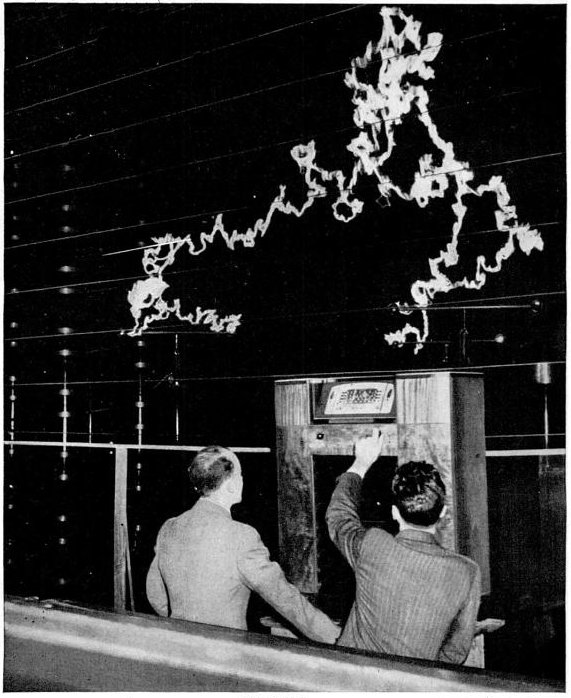FM具有比AM更强的抗噪声和RFI能力,在百万伏特电弧放电的环境下,AM接收机只能收到一片白噪声,而FM接收机却能清晰地解调出音乐(1940s, New York publicity demonstration by General Electric)

## 1.3 PM(Phase Modulation)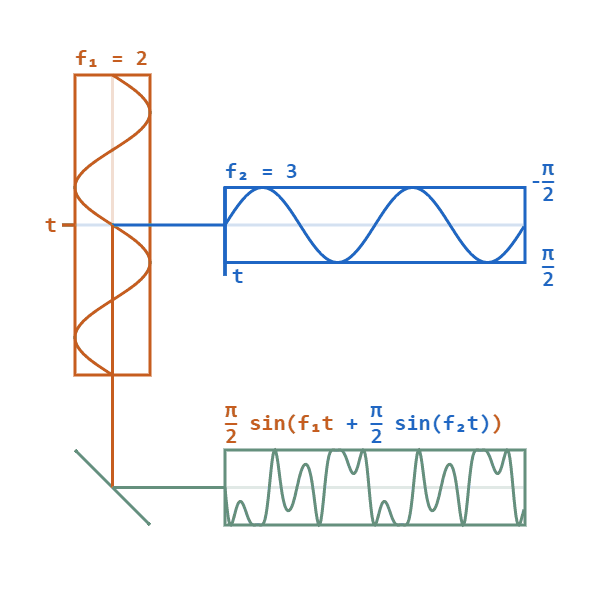### 1.3.1 数学形式

$c(t) = A_c \sin (\omega_c t + \phi_c)$

$y(t) = A_c \sin (\omega_c t + m(t) + \phi_c)$

$m(t) = \cos(\omega_c t + h\omega_m(t))$

PM的频谱结构表明有2个需要重点考虑的信号区域:

• 对于小幅度信号,PM非常类似于AM,但不幸的是PM的基带带宽增加了一倍,导致效率非常低。
• 对于单频大正弦信号,PM非常类似于FM,其带宽约为: $$2(h+1)f_M$$, $$f_M=\omega_m/2\pi,h$$是调制指数,这也被称为PM的卡森带宽法则。。

### 1.3.2 调制指数

$h = \Delta \theta$

$$\Delta \theta$$ 是最大相位变化量。

### 1.3.3 PM的性质

1. PM的传送功率具有恒定性,不难想象。
2. PM的调制过程的非线性性质 PM并不满足叠加性原理,此性质让PM的频谱分析与噪声分析变得非常复杂,但也因此,PM具有优异的抗噪声性能~
3. 零相交的不规律性 零相交(zero-crossing)是指，在时间轴上，波的幅度由正变成负或由负变成正的瞬间。而一个PM波的零相交并没有规律性存在。事实上，PM波的零相交不规律性，是因为调制过程的非线性性质所致。
4. 信息波形难以形象化 对于调幅，只要调制百分比小于百分之百，便可以将调制波的包络视为信息波。但是，PM的波形非常抽象...这也是因为第二条性质所致。
5. 可通过增加传输带宽来换取更好的噪声性能表现 和AM相比，相位调制一个非常好的优点是 它可以改善噪声性能。因为对于相加性噪声，调制相位比调制幅度受噪声的影响要小很多。
但是，PM噪声性能的改善是通过牺牲传输带宽来实现的。相比之下,AM不可能有这样的机会~

## 1.4 AM/FM/PM应用与对比(多图)

AM与FM在无线电广播中应用很普遍，例如我们耳熟能详的 86.6MHz FM广播,衬衫的价格是9磅15便士

AM与FM的频率划分各地略有不同。在中国,调幅(AM)广播频段为 525kHz-1605kHz频率段，这个频段被称为 中波。调频(FM)广播频段为 88MHz-108MHz,我们一般听的车载电台都是FM。市面上常见的收音机多为FM收音机,而AM收音机已经比较少了。但是如果您也是xidian学生的话，你的电装实习项目就是做一台纯模拟的AM收音机:)

AM No 很差,应用场景较少 载波频率不变,幅度跟随基带变化 可低可高
FM Yes 很好,是高频、高保真无线电传输的标准 载波幅度不变,频率跟随基带变化 相对较高
PM Yes 很好,在数字传输编码方案中应用广泛 载波幅度不变,相位跟随基带变化 相对较高

### 1.4.1 AM的实际波形与频谱

AM调制波形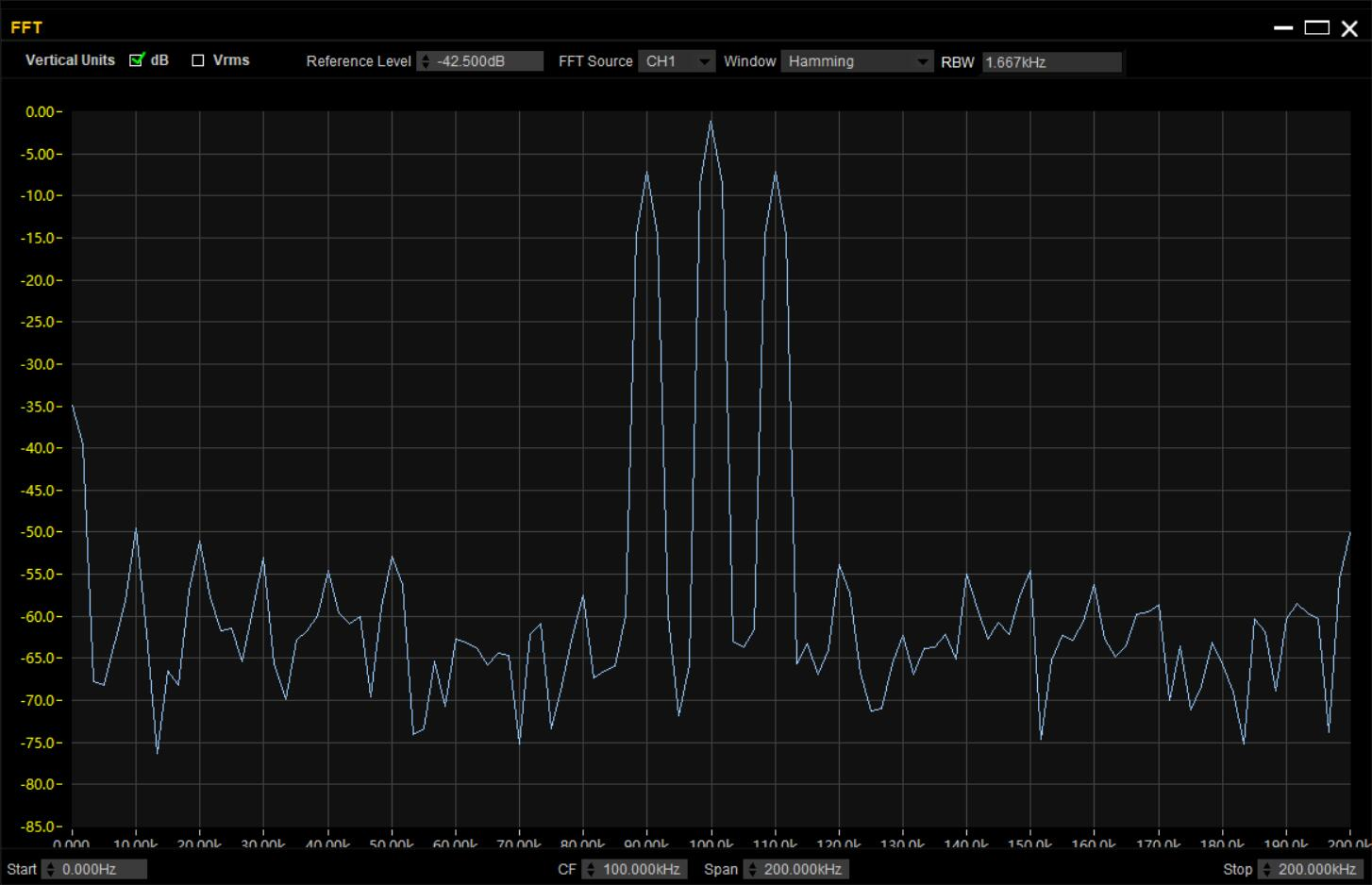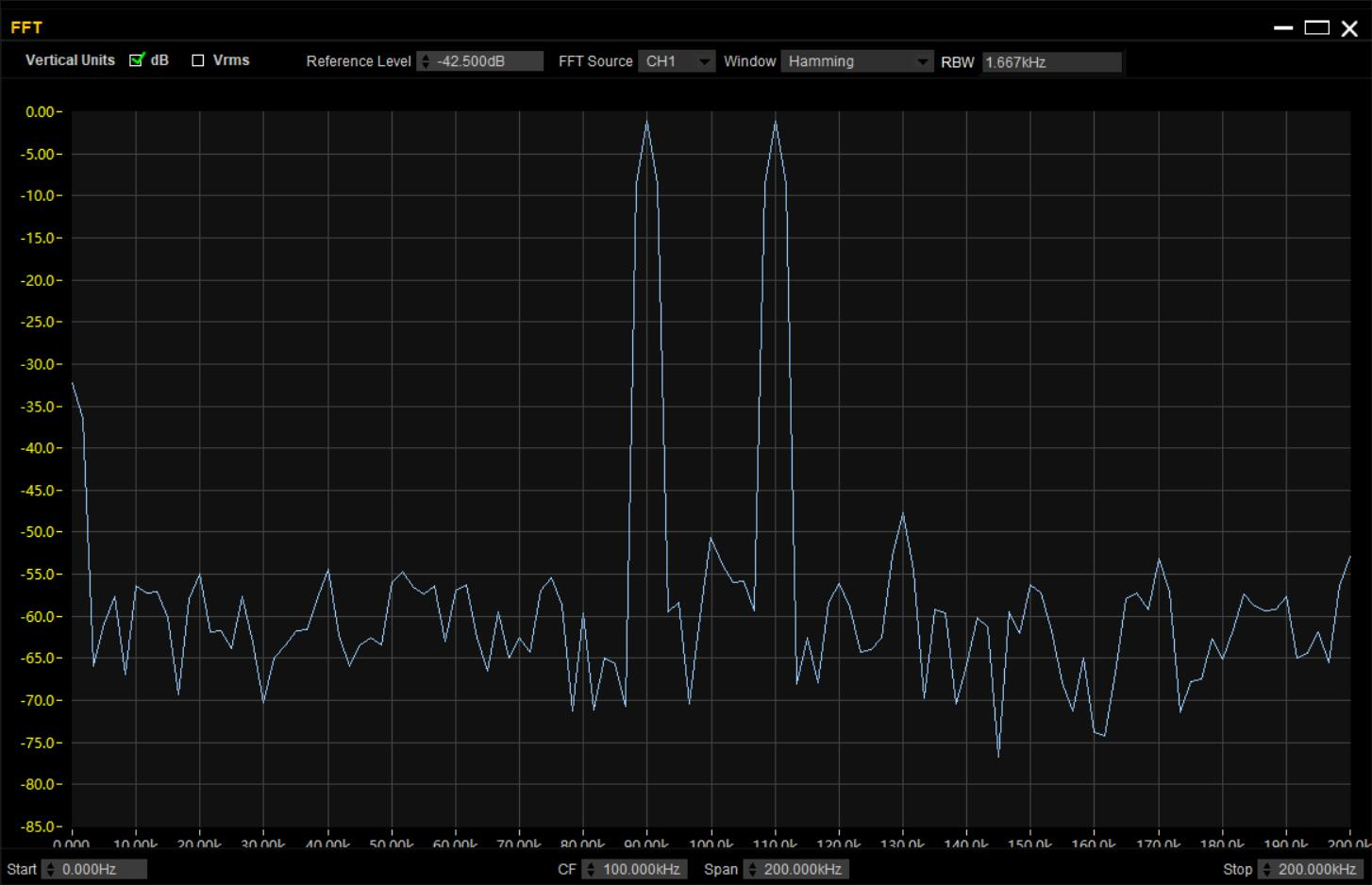### 1.4.2 FM的实际波形与频谱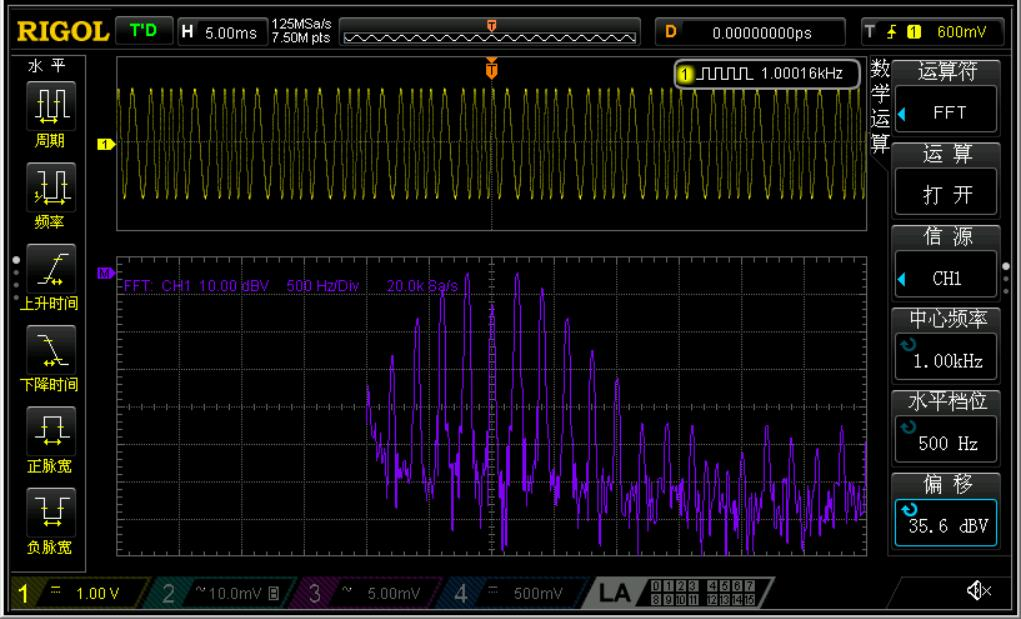### 1.4.3 PM的实际波形与频谱

PM的频谱,因为这台示波器的FFT深度不够,RBW太大了可能有些峰压根没分辨出来..所以凑合看看就行233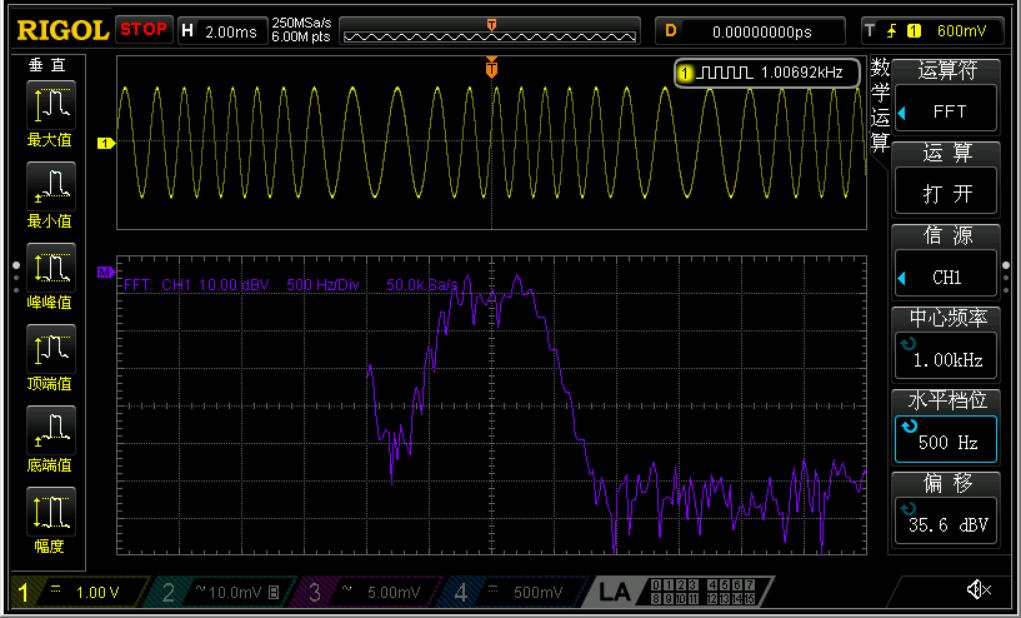3种模拟调制都港完了，我们马上开始进入数字时代！

# 二.数字调制

## 2.0 数字调制与模拟调制的区别• 模拟开关
• 压控增益放大器
• 继电器(划去) (开玩笑的,继电器打咩打咩。)
• 在数字域中实现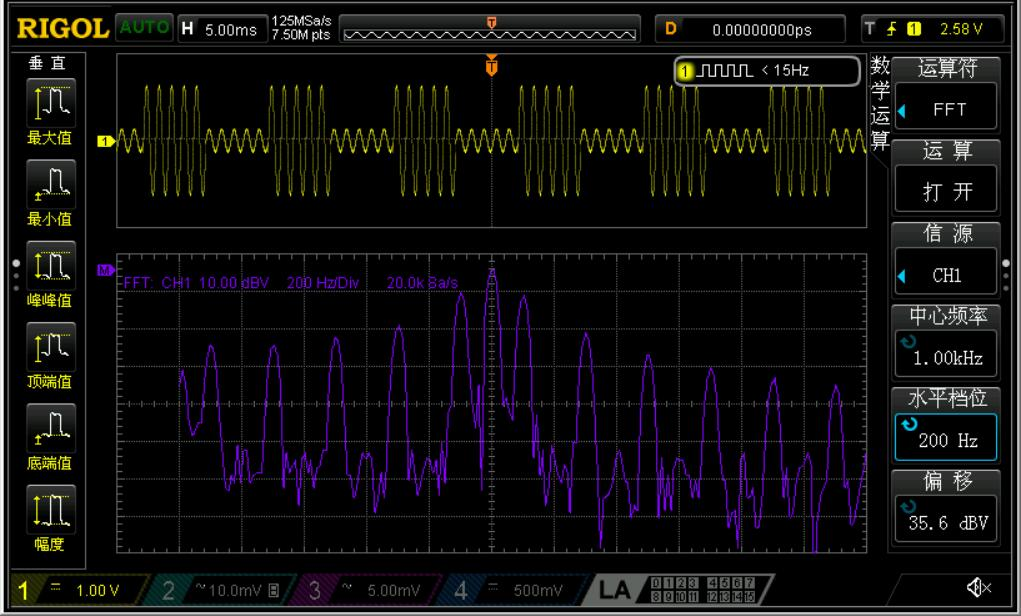## 2.2 FSK(Frequency-Shift Keying)### 2.2.1 FSK实现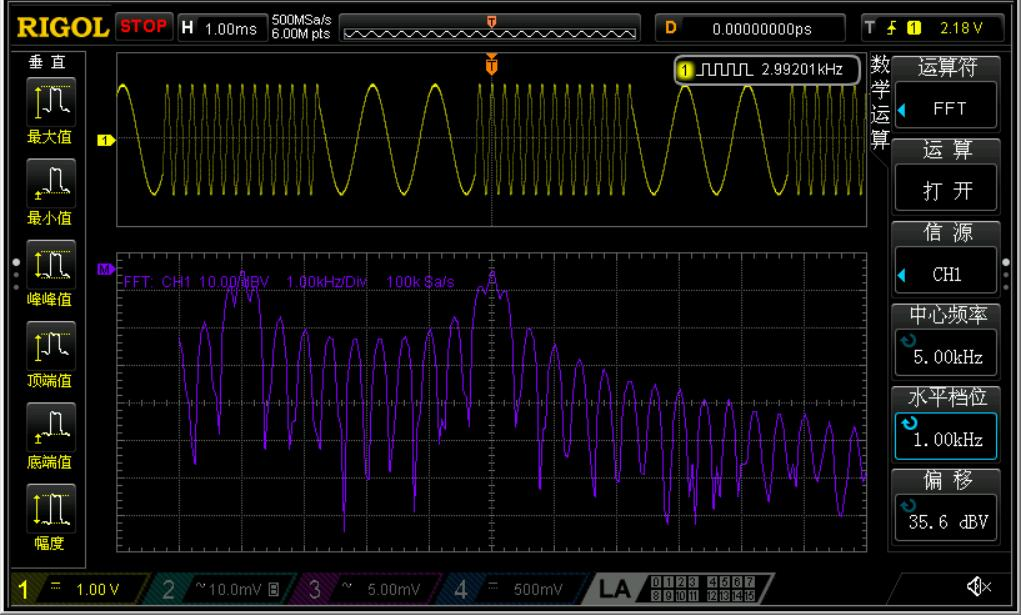## 2.3 PSK(Phase-Shift Keying)

PSK又可称为 M-PSKMPSK,M代表传送信号的 符号(symbol)种类。符号种类越多(M越大),传送的bits数越多,通信速率越高。比如说二进制PSK的M=2，一般称为BPSK或2PSK。相应的还有4进制(QPSK)、8PSK、16PSK等。但M-PSK会因M的提升而使得误码率上升,M大于16之后误码率(BER)就有点大到无法接受的程度了,所以16之后都由另一种高阶调制——QAM来执行调制工作。

M-PSK的误码率与信噪比的关系,可以看出在相同信噪比条件下,多符号种类会导致更高的误码率(BER)### 2.3.1 数学形式

$s_n(t) = \sqrt{2E_b \over T_b} \cos(2\pi ft + \pi (1-n)) \cdots (n = 0,1)$

$s_n(t) = \sqrt{2E_b \over T_b} \cos(2\pi ft + \pi) = -\sqrt{2E_b \over T_b} \cos(2\pi ft), \cdots binary "0" \\ s_n(t) = \sqrt{2E_b \over T_b} \cos(2\pi ft), \cdots binary "1"$

### 2.3.2 星座图(Constellation diagram)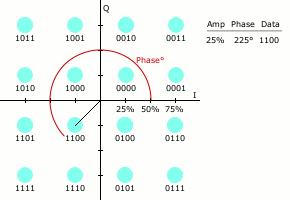### 2.3.4 Waveforms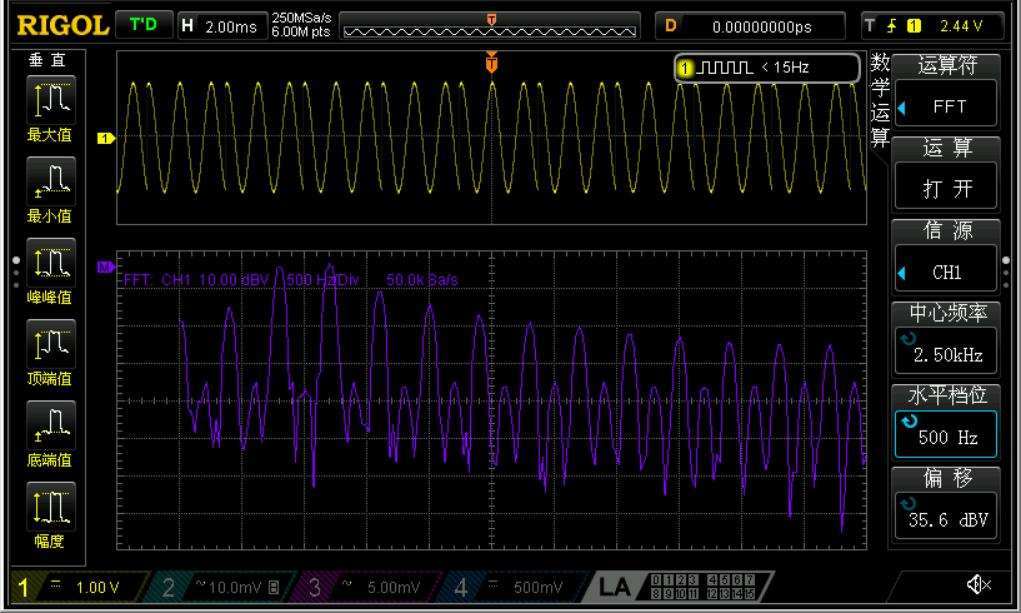# 三.特殊信号

## 3.1 Chirp(啁啾)信号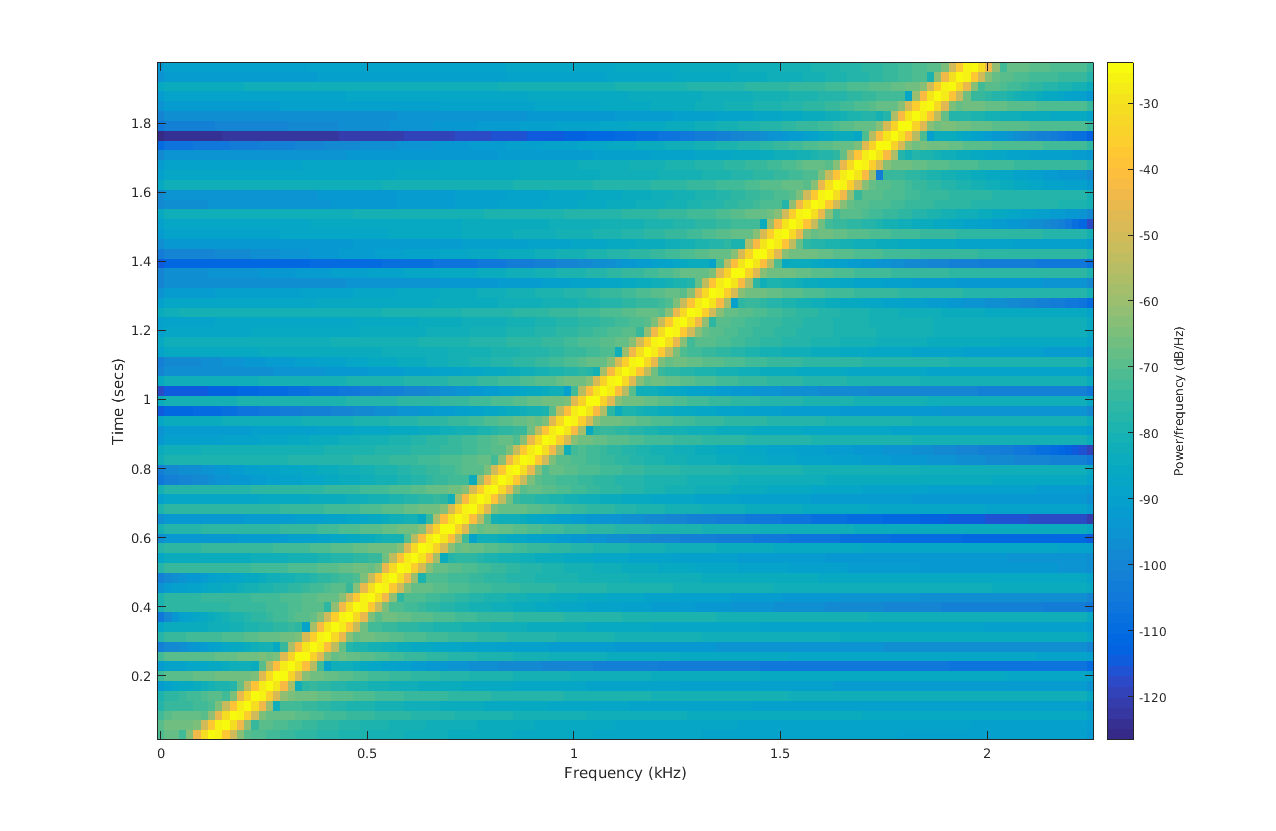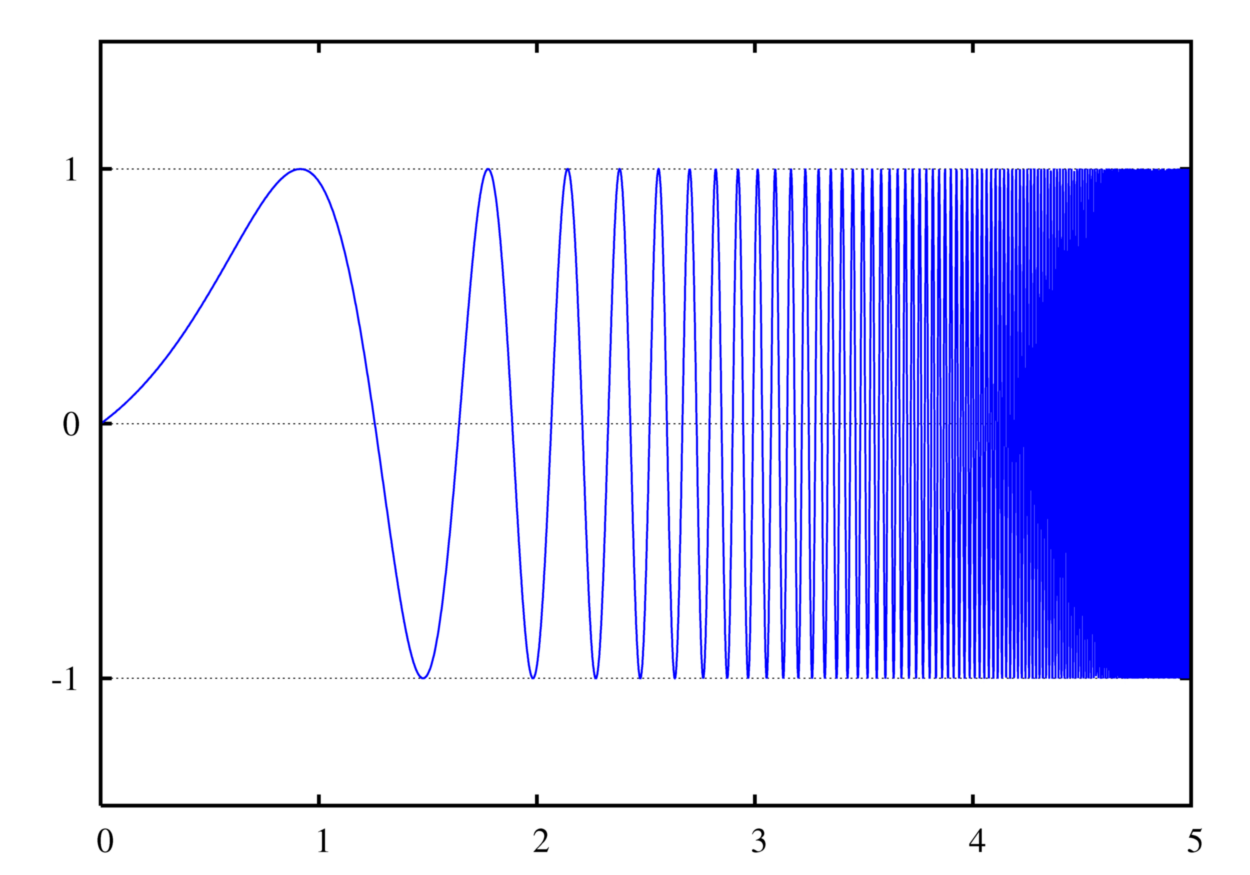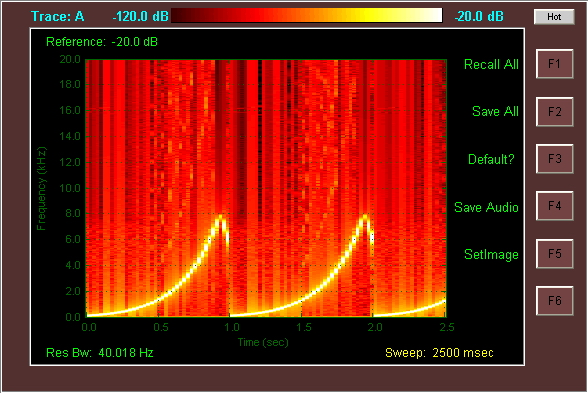### 3.1.1 Chirp信号的产生

1. 使用一锯齿波控制压控振荡器(VCO),以此来产生频率线性增长的Chirp信号
2. 在数字域中合成Chirp信号(在DSP中直接运算出波形数据),然后用DAC直接输出
3. 使用DDS器件,直接修改频率控制字(Frequency Tuning Word)

### 3.1.2 Chirp信号的用途

Chirp信号由于其跟脉冲信号的相关性(数学上)，非常适合用于无线电和声波探测技术中的 脉冲压缩 技术上。所以首先Chirp可用于雷达和声纳上,能够使这些系统测量长距离的同时又保持高时间分辨率。

# 五.推荐学习资料

PySDR - 利用Python交互式地学习通信原理与软件无线电(SDR)

I/Q Data for Dummies - 利用图形形象地解释什么是I/Q

Matlab Communication Toolbox - 通信工具箱

Think-DSP 这是一个Professor —— Allen Downey 写的一本电子教材,他旨在通过Python及其众多功能强大的库来呈现数字信号处理(DSP)的无限魅力...总之是计算机与EE完美结合的典范~